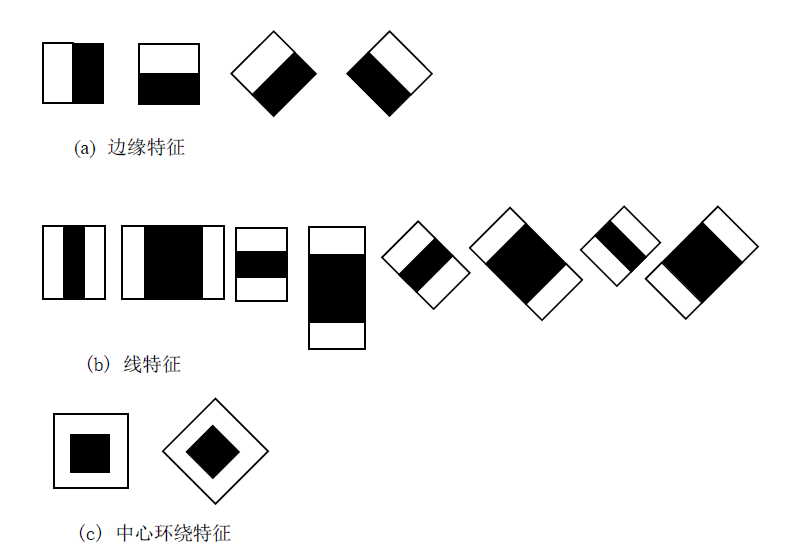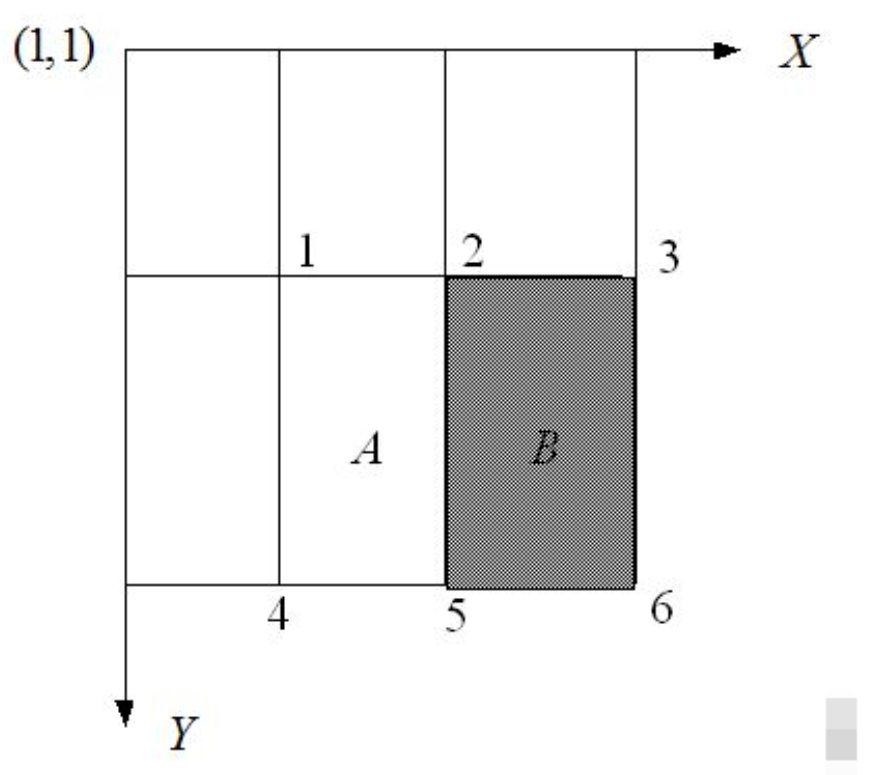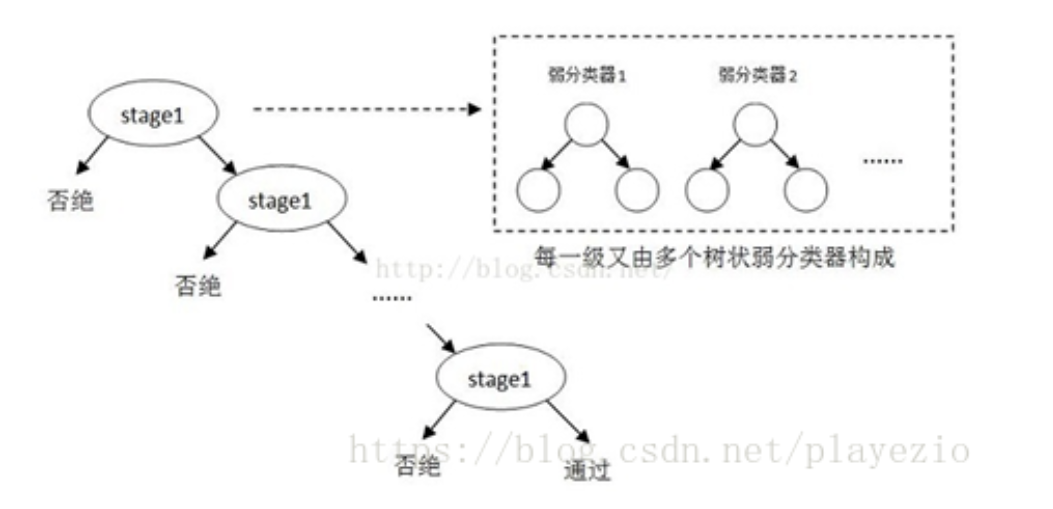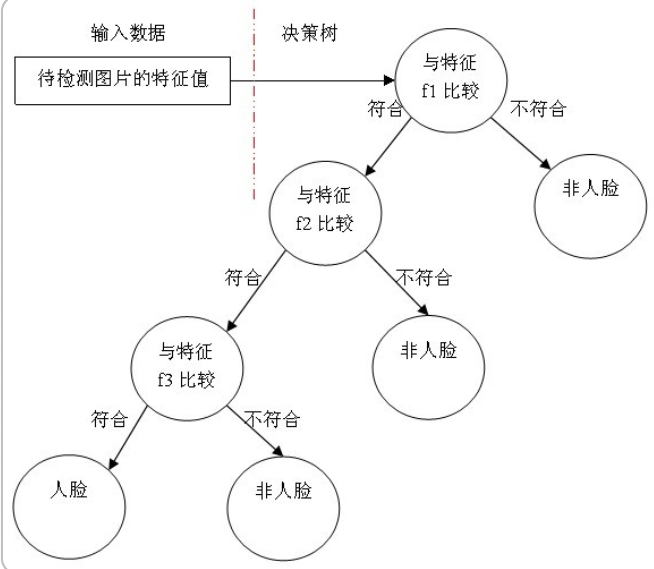# Haar特征描述算子与人脸检测

### 目录

• Haar-like 特征描述算子
• 特征类别（模板）
• 特征计算—积分图
• 计算Haar特征值
• Haar特征值归一化(方法不唯一)
• 级联分类器
• 级联分类模型
• 级联分类器的训练
• XML文件
• 程序

## 特征类别（模板）

Haar(哈尔)特征模板分为三类：边缘特征、线性特征（含对角线特征）、中心特征。其结构如下图所示。Haar特征值反映了图像的灰度变化情况。但矩形特征只对一些简单的图形结构，如边缘、线段较敏感，所以只能描述特定走向（水平、垂直、对角）的结构。

（1）如何快速计算那么多的特征？—积分图；

## 特征计算—积分图

$ii(i,j)=\sum_{k≤i,l≤j} f(k,l)$

1、用𝑠(𝑖,𝑗)表示行方向的累加和，初始化𝑠(𝑖,−1)=0；
2、使用𝑖𝑖(𝑖,𝑗)表示一个积分图像，初始化𝑖𝑖(−1,𝑖)=0；
3、逐行扫描图像，递归计算每个像素(𝑖,𝑗)行方向的累加和𝑠(𝑖,𝑗)和积分图𝑖𝑖(𝑖,𝑗)的值： $s(i,j)=s(i,j−1)+f(i,j)$

$ii(i,j)=ii(i−1,j)+s(i,j)$

4、扫描图像一遍，当到达图像右下角像素时，积分图像𝑖𝑖就构建好了。

## 计算Haar特征值## Haar特征值归一化(方法不唯一)

1、计算检测窗口中图像的灰度值和灰度值平方和： $sum=\sum i(x,y)$

$sq_{sum}=\sum i^2(x,y)$

2、计算平均值： $mean = \frac{sum}{w*h}$

$sq_{mean}=\frac{sq_{sum}}{w*h}$

3、计算归一化因子： $varNormFactor=\sqrt{sq_{mean}-mean^2}$ 4、归一化特征值： $normValue=\frac{featureValue}{varNormFactor}$ 之后使用归一化的特征值𝑛𝑜𝑟𝑚𝑉𝑎𝑙𝑢𝑒与阈值对比。

（2）OpenCV自带的XML分类器中各项参数，如internalNodes和leafValues标签里面的一大堆数字的意义。

## 级联分类器

### 级联分类模型### 级联分类器的训练

1.训练原理$h(x,f,ρ,Θ)=\begin {cases}1, {ρf(x)<ρΘ}\\ 0, {other}\end{cases}$

2.训练步骤

1、对于每个特征 𝑓，计算所有训练样本的特征值，并将其排序：

2、扫描一遍排好序的特征值，对排好序的表中的每个元素，计算下面四个值：

3、选取当前元素的特征值$F_{k,j}$和它前面的一个特征值$F_{k,j-1}$之间的数作为阈值，这个阈值对应的弱分类器将当前元素前的所有元素分为人脸（或非人脸），而把当前元素后（含）的所有元素分为非人脸（或人脸）。

1.、给定训练样本集$\left(x_{i}, y_{i}\right), i=1,2,3, \ldots N$,共N个样本，$y_i$取值为0(负样本)或者1(正样本)；设人脸正样本的数量为$n_1$，负样本数量为$n_2$； T为训练的最大循环次数；

2.、初始化样本权重为$\frac{1}{n_1+n_2}$，即为训练样本的初始概率分布；

3、$for\ t=1,...T$:
①权重归一化 $ω_{t,i}=\frac{ω_{t,i}}{\sum\limits_{j-1}^{n}ω_{t,j}}$

②对每个(种)特征$f_j$，训练一个弱分类器$h_j$，每个分类器只使用一种Haar特征进行训练。分类误差为： $ε_j=\sum\limits_{i}ω_i|h_j(x_i)-y_i|$

③从②确定的弱分类器中，找出一个具有最小分类误差的弱分类器$h_t$;

④更新每个样本对应的权重: $\omega_{t+1, i}=\omega_{t, i} \beta_{t}^{1-e_{i}}$

3、级联分类器的检测

1、准备人脸、非人脸样本集；
2、计算特征值和积分图；
3、筛选出T个优秀的特征值（即最优弱分类器）；
5、级联。

## XML文件

OpenCV 自带了训练器和检测器。这里我们介绍的XML文件，就是OpenCV自带的检测器。

(1)使用python pip安装最新的opencv-python包：pip install opencv-python
(2)查看包的位置：pip show opencv-python image-20200614115759788
（3）进入红框的文件夹：cd d f:\cjc\imagefusion\venv\lib\site-packages（根据上一步结果选择）

（4）进入这个文件夹下的cv2目录：cd cv2
XML文件就在data/文件夹下

xml文件主要保存相关的特征矩阵，以及各个弱分类器相关的信息。

## 程序

import cv2
import numpy as np

# 1.静态图像中的人脸检测
def StaticDetect(filename):
# 创建一个级联分类器 加载一个 .xml 分类器文件. 它既可以是Haar特征也可以是LBP特征的分类器.

# 加载图像
# 转换为灰度图
gray_img = cv2.cvtColor(img, cv2.COLOR_BGR2GRAY)
# 进行人脸检测，传入scaleFactor，minNegihbors，分别表示人脸检测过程中每次迭代时图像的压缩率以及
# 每个人脸矩形保留近似数目的最小值
# 返回人脸矩形数组
for (x, y, w, h) in faces:
# 在原图像上绘制矩形
img = cv2.rectangle(img, (x, y), (x + w, y + h), (255, 0, 0), 2)
cv2.namedWindow('Face Detected！')
cv2.imshow('Face Detected！', img)
cv2.waitKey(0)
cv2.destroyAllWindows()

# 2、视频中的人脸检测
def DynamicDetect():
'''
打开摄像头，读取帧，检测帧中的人脸，扫描检测到的人脸中的眼睛，对人脸绘制蓝色的矩形框，对人眼绘制绿色的矩形框
'''
# 创建一个级联分类器 加载一个 .xml 分类器文件. 它既可以是Haar特征也可以是LBP特征的分类器.

# 打开摄像头
camera = cv2.VideoCapture(0)
cv2.namedWindow('Dynamic')

while True:
# 读取一帧图像
# 判断图片读取成功？
if ret:
gray_img = cv2.cvtColor(frame, cv2.COLOR_BGR2GRAY)
# 人脸检测
for (x, y, w, h) in faces:
# 在原图像上绘制矩形
cv2.rectangle(frame, (x, y), (x + w, y + h), (255, 0, 0), 2)
roi_gray = gray_img[y:y + h, x:x + w]
# 眼睛检测
eyes = eye_cascade.detectMultiScale(roi_gray, 1.03, 5, 0, (40, 40))
for (ex, ey, ew, eh) in eyes:
cv2.rectangle(frame, (ex + x, ey + y), (x + ex + ew, y + ey + eh), (0, 255, 0), 2)

cv2.imshow('Dynamic', frame)
# 如果按下q键则退出
if cv2.waitKey(100) & 0xff == ord('q'):
break

camera.release()
cv2.destroyAllWindows()

if __name__ == '__main__':
filename = 'face.jpg'
StaticDetect(filename)
#DynamicDetect()


### 热门文章## PAT 1161 Merging Linked Lists## 报表热切换是什么意思？如何做到？## 【考试记录】Apsara Clouder基础技能认证：实现调用API接口## leetcode 718.最长重复子数组## 软件工程复习## Java报SSL的错误：javax.net.ssl.SSLException MESSAGE: closing inbound before receiving peer‘s close_notify## win10系统Idea运行maven项目的编码设置## mongoDB采坑

mongoDB采坑 安装问题没有权限参考 https://blog.csdn.net/qq_20084101/article/details/82261195## Autosar4.4：通用架构模板 - 元建模模式与模型转换（2/3）## 参考nacos写的Registry注册中心

Registry注册中心 github链接:https://github.com/lzj-github/registry 麻烦大家顺便点个star，谢谢啦！ 在学习了SpringCloud的各个组件的使用以及研究过相关源码后，为了更好地理解其内部实现原理，模仿了nacos的部分设计思想，自己实现了该注册中心，代码量4000+，代码中有详…## jvm垃圾收集算法以垃圾收集器简介

jvm垃圾收集算法以垃圾收集器简介 每天多学一点点~ 话不多说，这就开始吧… 文章目录jvm垃圾收集算法以垃圾收集器简介1.前言2.内存模型判断机制3.垃圾收集算法3.1 分代收集理论3.2 标记-复制算法3.3 标记-清除算法3.4 标记-整理算法4.常用的垃圾收集器4.1 Serial收集器(-XX:+U…## LeetCode 226. 翻转二叉树## JS Array## reid常用评价指标roc rank1 map,误识率far, 以及optim lr_scheduler 学习率衰减函数

1 roc, 人脸识别或者reid问题 误识率far *roc 横坐标是 误识率 far(fpr） 纵坐标是tpr * ROC曲线-阈值评价标准 https://blog.csdn.net/abcjennifer/article/details/7359370 用来确定不同数据集下的阈值，实际情况下的不同数据集 由于是在没有label下的查找， 需要限定阈值…## 共享内存实现多进程低延迟队列 10us## 「bitset」库下的便捷二进制转换## web安全专题（1）注入攻击## 初级Java工程师也能轻松进行JVM调优了## JS奇淫巧技

0. 向下取整最快方式 向下取整有很多方法, Math.floor, parseInt都可以, 不过两个非(~)运算符来取整是最方便的, 而且逻辑运算很快.还可以用~~再加1来向上取整.~~3.14 > 3 ~~Math.PI > 3 ~~Math.E > 2 ~~12.98 > 12var b= 2.33 | 0 ---b=2var c= 2.33 >> …